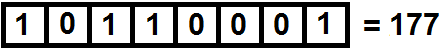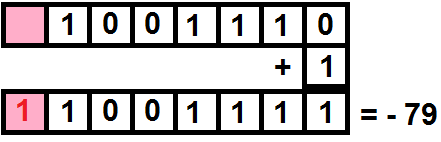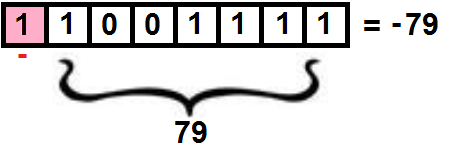# Two mobiles. Bluetooth Server - Client. Study

Hello friends,

• I wrote two topics about Server-Client mobile connections via Bluetooth:

Now I am going to write a little tutorial on the data types sent.

Here tutorial in Spanish:
http://kio4.com/appinventor/9C3_bluetooth_servidor_cliente.htm

• First we connect the Server, then the Client. When they are connected, click on "Start Clock" to start receiving data

1.--

• We can send a Text: "El ñandú tiene 46 años." and receive it as a text on the Client.

• We can also receive that text as UnsignedBytes, we will obtain a list with the ASCII value of each character:

[69, 108, 32, 195, 177, 97, 110, 100, 195, 186, 32, 116, 105, 101, 110, 101, 32, 52, 54, 32, 97, 195, 177, 111, 115 , 46]

• If we receive it as SignedBytes, those numbers greater than 127, we will receive them in negative.

[69, 108, 32, -61, -79, 97, 110, 100, -61, -70, 32, 116, 105, 101, 110, 101, 32, 52, 54, 32, 97, -61, -79, 111, 115, 46]

2.--

• We can also send a list of Bytes with decimal values, for example: 65, 66, 67, 68, 69

• If we receive them as text, we will obtain their ASCII character, in our case we will receive: ABCDE

• If we receive them as UnsignedBytes, we will obtain a list with the numerical values ​​sent:
[65, 66, 67, 68, 69]

• If we receive them as SignedBytes, this time we will obtain the same previous values, since none exceeds 127

3.--

• Another possibility is to send the information using 4 Bytes Number, this must be a decimal number from 0 to 4,294,967,296 (256 x 256 x 256 x 256)

• In the example we send: 3,999,368,245 we will receive it as UnsignedBytes in list form:
[53, 132, 97, 238]

• It is a binary number encoded in decimal parts, whose decimal value is:

53 x 1 + 132 x 256 + 95 x 65,536 + 238 x 16,711,580 = 3,999,368,245

• If we receive it as SignedBytes, we will obtain:
[53, -124, 97, -18]

• Negative numbers are due to exceeding 127.

3.- Two's Complement. Signed numbers.

• We have the number 177, we convert it to binary and we obtain:• If we convert that number to signed binary, it turns out that the left bit becomes a sign and the numerical value corresponds to the two's complement of the other 7 bits.

• Two's complement, it results from inverting the bits and adding a 1 to the number obtained:• We make this change when the number we want to convert is greater than 127.

• One way to obtain the decimal number is to subtract it from 256, that is

256 - 177 = 79